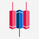Long

# LTCUSD Update: Profit=69525 SL=6310 Reward/Risk > 11:1

BITSTAMP:LTCUSD   Litecoin / U.S. Dollar
432 views

Profit targets=69525 pip (690+ 1230+ 1960+ 3060+ 4085 + 6070+ 7505+ 10280+ 15280+ 19365)
Stop Loss =6310 pip
Reward/Risk > 11: 1

Current Status:

Total Profit: 7508 pip

We opened 11 BUY trade(s) @ 47.49 based on 'Valley' entry method at 2020.07.25.

Closed Profit:
TP1 @ 50.65 touched at 2020.07.26 with 316 pip Profit.
TP2 @ 56.05 touched at 2020.07.28 with 856 pip Profit.
316 + 856 = 1172

Open Profit:
Profit for one trade is 54.53(current price) - 47.49(open price) = 704 pip
9 trade(s) still open, therefore total profit for open trade(s) is 704 x 9 = 6336 pip

Technical analysis:
. LTCUSD is in a range bound and the beginning of uptrend is expected.
. The price is above the 21-Day WEMA which acts as a dynamic support.
. The RSI is at 72.

* There is still a possibility of temporary retracement to suggested support line (48.75). if so, traders can set orders based on Price Action and expect to reach short-term targets.
* If you missed our first HUNT, you have a second chance to buy above the suggested support line (48.75).

Take Profits:
TP1= 50.65
TP2= 56.05
TP3= 63.35
TP4= 74.35
TP5= 84.60
TP6= 104.45
TP7= 118.80
TP8= 146.55
TP9= 196.55
TP10= 237. 40
TP11= Free

❤️ If you find this helpful and want more FREE forecasts in TradingView
. . . . . . . . Hit the 👍 LIKE button,
. . . . . . . . . . . Drop some feedback below in the comment!

❤️ Your Support is very much 🙏 appreciated!❤️

Be sure to leave a comment let us know how do you see this opportunity and forecast.

ForecastCity English Support Team ❤️

Total Profit: 11886

Update Details:

Closed Profit:
TP1 @ 50.65 touched at 2020.07.26 with 316 pip Profit.
TP2 @ 56.05 touched at 2020.07.28 with 856 pip Profit.
TP3 @ 63.35 touched at 2020.08.02 with 1586 pip Profit.
316 + 856 + 1586= 2758

Open Profit:
Profit for one trade is 58.90(current price) - 47.49(open price) = 1141 pip
8 trade(s) still open, therefore total profit for open trade(s) is 1141 x 8 = 9128 pip
All SLs moved to Break-even point.

Take Profit:
TP4= 74.35
TP5= 84.60
TP6= 104.45
TP7= 118.80
TP8= 146.55
TP9= 196.55
TP10= 237.40
TP11= Free
HighAccurate forecast for
★36 Forex Pairs: 750,000 pips track record profit
★34 Cryptos
★Gold, Silver, Oils, Gas

in 8 languages

since 2007

http://www.ForecastCity.com
★FREE Telegram http://t.me/forecastcity_com